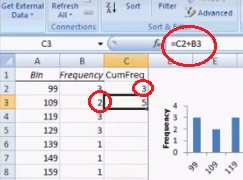# Cumulative Frequency Table in Excel: Easy Steps

Share on

Probability and Statistics > Microsoft Excel for Statistics > Cumulative Frequency Table in Excel

In order to follow this article, you should know how to create a regular distribution table/histogram in Excel. Don’t know how? Check out this video/article first: How to Make a Frequency Distribution Table in Excel.

Watch the video to find out how to make a Cumulative Frequency Table in Excel:

## How to Make a Cumulative Frequency Table in Excel: Steps

Step 1: Create a regular frequency distribution table in an Excel worksheet (see: How to Make a Frequency Distribution Table in Excel.)

Step 2: Add a third column to your frequency chart. Title it “Cumulative Frequency.”

Step 3: Type the formula “=B2” (where B2 is the actual location of your first frequency count) in the first row of your new column.

Step 4: Type the formula “=C2+B3” (where C2 is the actual location of your first cumulative frequency count from Step 3, and B3 is the location of your second frequency count) in the first row of your new column.
If this step sounds a little confusing (why add one cell from each column instead of adding down the row?), that’s because you’re setting up Excel to enter formulas automatically. Follow along the next step and you’ll see why the formula is set up that way.

Step 5: Click the cell you entered the formula in Step 4. Click and drag the little black square in the bottom right hand corner of the cell to the bottom of the column. Excel will populate the cell with all of the remaining values.

That’s it!

Check out our YouTube channel for hundreds more statistics videos!

CITE THIS AS:
Stephanie Glen. "Cumulative Frequency Table in Excel: Easy Steps" From StatisticsHowTo.com: Elementary Statistics for the rest of us! https://www.statisticshowto.com/cumulative-frequency-table-excel-easy-steps/
---------------------------------------------------------------------------Need help with a homework or test question? With Chegg Study, you can get step-by-step solutions to your questions from an expert in the field. Your first 30 minutes with a Chegg tutor is free!